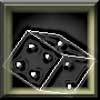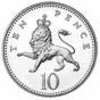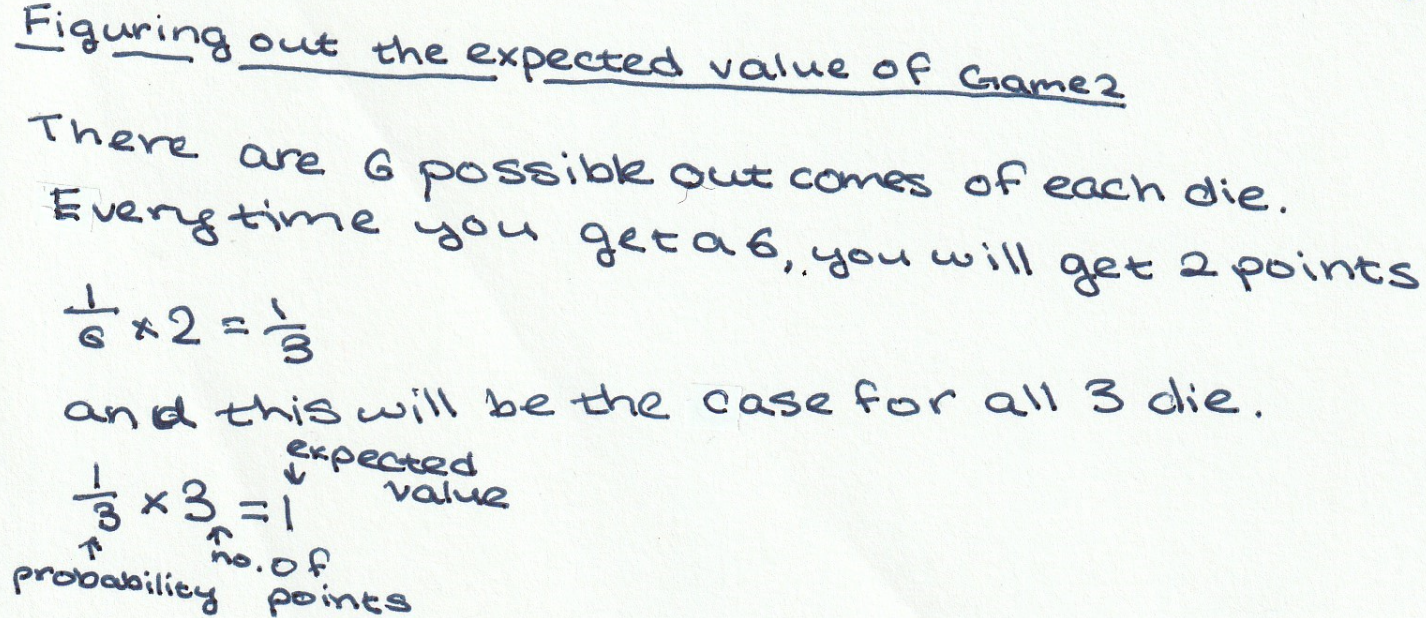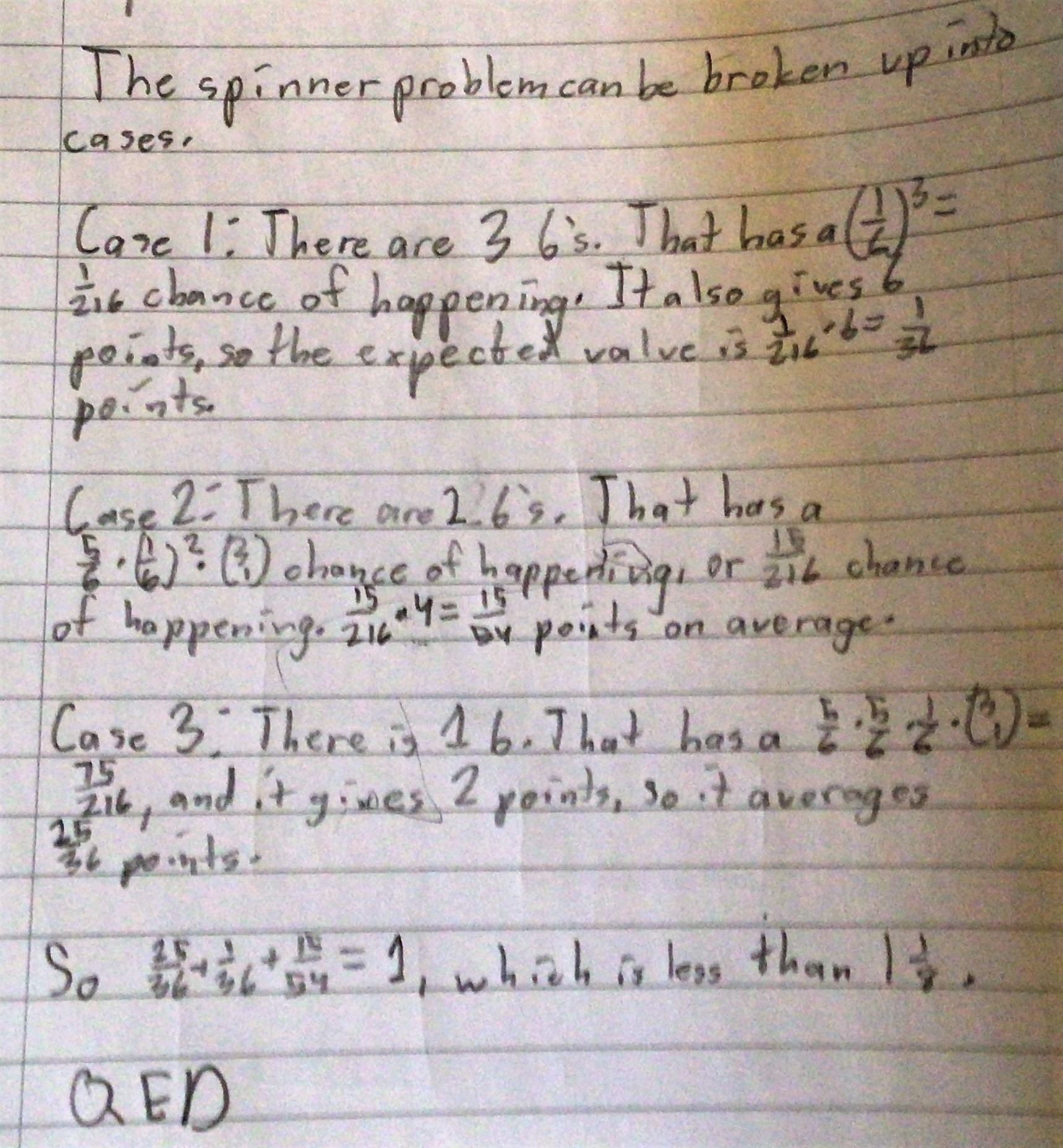#### You may also like### Gambling at Monte Carlo

A man went to Monte Carlo to try and make his fortune. Is his strategy a winning one?### Marbles and Bags

Two bags contain different numbers of red and blue marbles. A marble is removed from one of the bags. The marble is blue. What is the probability that it was removed from bag A?### Coin Tossing Games

You and I play a game involving successive throws of a fair coin. Suppose I pick HH and you pick TH. The coin is thrown repeatedly until we see either two heads in a row (I win) or a tail followed by a head (you win). What is the probability that you win?

# The Better Choice

##### Age 14 to 16Challenge Level

We received many messages about this problem.  Solutions arrived from students attending schools and colleges worldwide, including Wilson's School and St Paul's School in the UK, Lancing College, Zydus School in India, Heckmondwike Grammar School, PSBBMS in India and the Frederick Irwin Anglican School in Australia. We also received a solution from Scout, but do not know their school.

Well done to everyone who submitted a solution explaining which game you would choose, we enjoyed exploring your mathematical thinking and reading about your investigations using the interactivities.

Zavya and Jasmine, from Heckmondwike, submitted their ideas after 'multiple tries' exploring our interactivity:

We came to the conclusion that Game 1 had a higher probability as opposed to Game 2.

Most, but not all of you, agreed with them that Game 1 should be viewed as the better choice.

Here are some thoughts from Tessa and Elizabeth from Frederick Irwin:

The best choice to play would be game one because the chances are higher for flipping two heads and tails than spinning a 6 on a spinner. We used the simulator and flipped and spun 100 times and the coins had more points.

Bella, also from Frederick Irwin, also thought that Game 1 was the better choice:

You would go with the coins. Since the coins have 3 points and the spinners have 2 points, the coins are more likely to get a higher score than the spinners. You also need to consider the probability of getting the right requirements to actually get the points. With the spinners, you need to get at least one 6 to gain any points, and there are 6 numbers per spinner that the arrow could land on, and there are 3 spinners. Whereas with the coins, there are only 2 sides per coin and there are 4 coins, so it is more likely that you will get points on the coins than the spinners.

Kushal from Hymers College in the England considered what would happen after a large number of games.

Game 1: Coins
chance of getting a specific sequence $=\frac12\times\frac12\times\frac12\times\frac12=\frac1{16}$
possible 2 heads 2 tail combinations = HHTT, HTHT, HTTH, TTHH, THTH, THHT = 6 combinations
$6 \times \frac1{16} = \frac38$ so chance of getting 2H and 2T is $\frac38$
$\frac{3}{8}$ of 100 = 37.5 wins
decimal wins arent possible, so it is 75 wins per 200 rounds
1 win is 3 points, 75$\times$3 = 225
so on average, you will get 225 points every 200 rounds

Game 2: Spinner
chance of getting a 6 = $\frac16$
we get 3 spins per round, in other words, for 100 rounds we have 300 spins
300 $\times\frac16$ = 50 sixes will be rolled in 100 rounds
one six gets 2 points
50$\times$2 = 100
so every 100 rounds is 100 points
double it for easy comparison:

Spinner = 200 points per 200 rounds
Coin flip = 225 points per 200 rounds
Playing the coins game will get you more points on average.

Keya from Zygus School in India, Homare from Wimbledon High School in the UK, David from the UK, Eric from Lakerigde in the USA and Sanika N from PSBBMS in India used a similar idea to work out the average win from game 1. This is Keya's work:

So in game 1, the total number of possible outcomes will be 16. The probability of getting two heads and two tails will be $\frac38$. Therefore, the number of points earned on each round will on average be ${3\times\frac{3}{8}}={\frac{9}{8}}$

Homare did the same thing for game 2 (talking about dice, rather than spinners, but the maths is the same):David's work did the same maths but used statistical notation. Click here to see David's work.

Eric and Sanika both used a longer method to get the same result for Game 2. This is Eric's work:Sanika's work is longer and considers each spinner separately. Click here to see Sanika's work on the coins and the spinners, including an exploration of what happens if it costs one point to play each game.